Function Repository Resource:

# FindLinearRecurrenceEquations

Find equations describing a linear recurrence corresponding to an input sequence

Contributed by: Max Piskunov and Jan Mangaldan
 ResourceFunction["FindLinearRecurrenceEquations"][list,a[n],n] attempts to find equations describing the minimal linear recurrence a[n] that generates list. ResourceFunction["FindLinearRecurrenceEquations"][list,a[n],n,d] attempts to find equations describing the linear recurrence a[n] of maximum order d that generates list. ResourceFunction["FindLinearRecurrenceEquations"][list] represents the equations in terms of formal symbols a and n.

## Details and Options

ResourceFunction["FindLinearRecurrenceEquations"] gives the equation for the recurrence itself, and the equations for its initial conditions.

## Examples

### Basic Examples (2)

Find recurrence equations for the Fibonacci sequence:

 In:=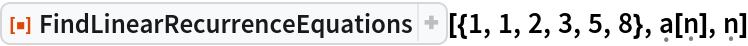Out=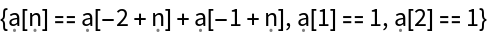Use the default symbols for the recurrence equations:

 In:=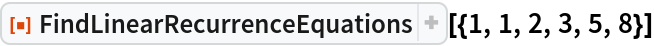Out=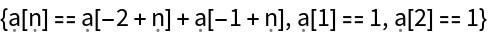A more complicated example:

 In:=Out=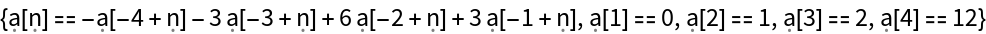### Scope (2)

Use symbolic data:

 In:=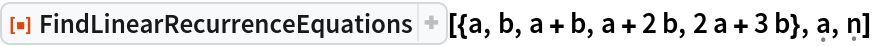Out=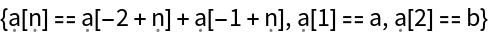Use the default formal symbols to represent the equations:

 In:=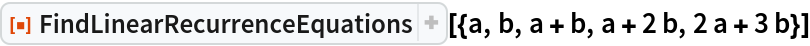Out=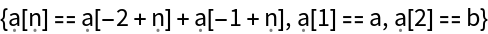Use different symbols for the equations:

 In:=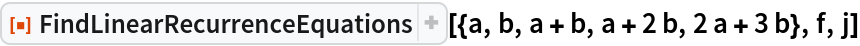Out=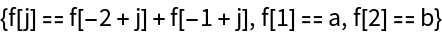In:=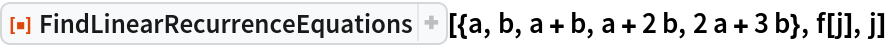Out=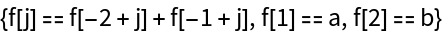### Applications (4)

Generate the convergents of a quadratic irrational:

 In:=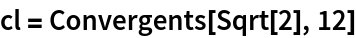Out=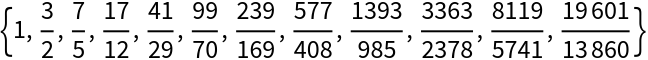Find the recurrence equations for the numerators and denominators:

 In:=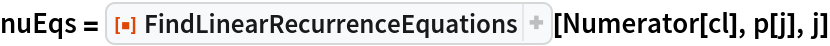Out=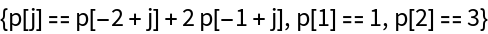In:=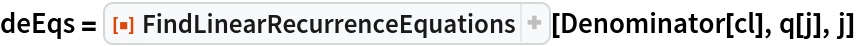Out=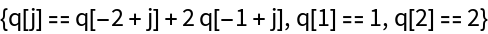Solve the two sets of recurrence equations:

 In:=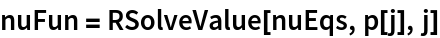Out=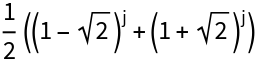In:=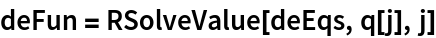Out=Verify that the limit at infinity of the ratio of the two solutions is the starting quadratic irrational:

 In:=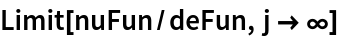Out=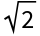### Properties and Relations (4)

Here are the first few values of the Padovan sequence:

 In:=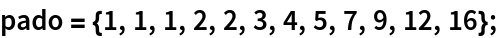Generate the recurrence equation and initial conditions with FindLinearRecurrenceEquations:

 In:=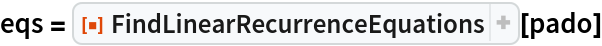Out=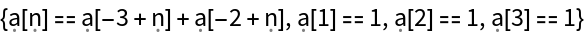Use RSolve to generate the explicit solution:

 In:=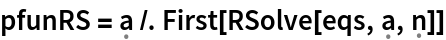Out=In:=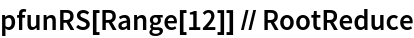Out=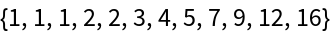Use DifferenceRoot to generate the implicit solution:

 In:=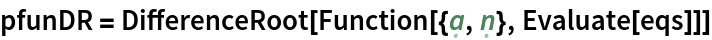Out=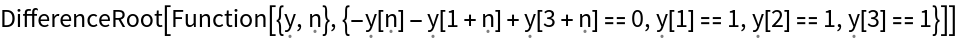In:=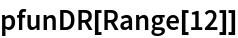Out=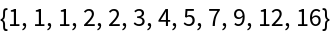## Version History

• 1.0.0 – 14 April 2020